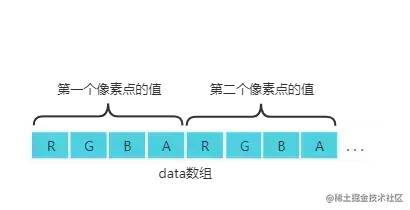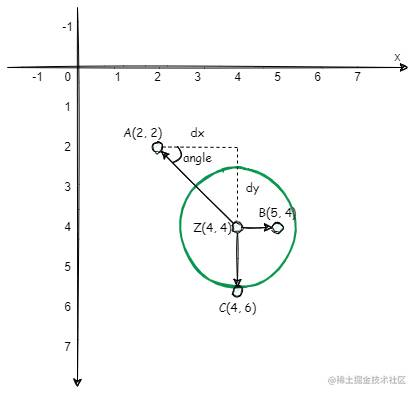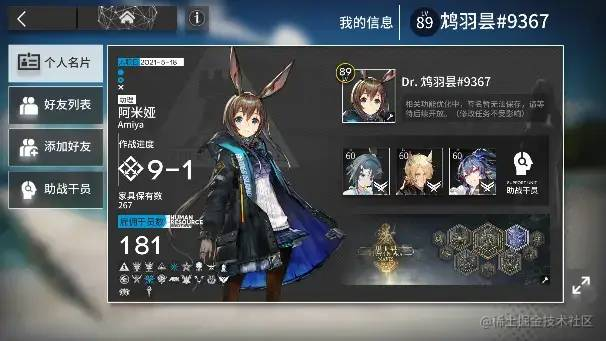# 【Canvas实战】仿明日方舟 Logo 粒子动画

## 最终效果

### 源码

github.com/XIwE1/ark-p…

1. Particle：记录粒子位置、颜色、大小、动画耗时 和 x/y 方向上的移动速度，提供绘制粒子方法`draw`、更新方法`update`、替换方法`change`
2. LogoImg：记录图片解析后的粒子数组信息`particleData`
3. ParticleCanvas：记录目标画布、画布中的粒子数组和鼠标在画布中的位置，提供绘制画布方法`drawCanvas`、改变粒子数组方法`changeImg`

• 实例化一个`ParticleCanvas`对象`prtCanvas`

• 点击某个图片`clickLogo`时调用`prtCanvas.changeImg(particleData)`方法传入其粒子数组信息。

• 首次 changeImg，直接赋值

• 非首次，对比粒子数组 移除/生成粒子，并随机映射

• 鼠标在实例对象`prtCanvas`对应的画布移动时触发`mousemove`回调，根据回调参数重新计算鼠标位置`mouseX/mouseY`

• `prtCanvas`的绘制画布方法`drawCanvas`一直随着事件循环在执行，`drawCanvas`中遍历画布粒子数组并调用每一项的`update`方法并传入重新计算后的`mouseX/mouseY`

• `particle.update`中又根据距离和设置好的引力/斥力重新计算`vx/vy`...

``````this.ParticleArr.forEach((particle) => {
particle.update(this.mouseX, this.mouseY);
particle.draw();
});

Particle 的 draw 方法符合面向对象的写法是接收一个 content 上下文参数，图方便就直接读取了

## 分析

1. 解析图片转换为粒子
2. 绘制时添加动画
3. 根据鼠标位置对粒子进行排斥

## 像素操作

canvas提供了 绘制图片 和 获取图片像素 的方法，但在绘制图片或者获取图片信息用于操作之前，首先要获取目标图片源

``````  ......
let img = new Image();
img.src = src;
// canvas 获取粒子位置数据
// 获取图片像素数据
const tmp_canvas = document.createElement("canvas"); // 创建一个空的canvas
const tmp_ctx = tmp_canvas.getContext("2d");

tmp_ctx?.drawImage(img, 0, 0, imgW, imgH); // 将图片绘制到canvas中
const imgData = tmp_ctx?.getImageData(0, 0, imgW, imgH).data; // 获取像素点数据
tmp_ctx?.clearRect(0, 0, width, height);
};
......

`ImageData``data`属性为 Uint8ClampedArray 类型的一维数组，包含了指定区域里每个像素点的RGBA格式的整型数据，范围在0至255之间（包括255）。## canvas动画

canvas的动画主要是通过 在一些定时方法中去执行重绘操作实现的。

canvas实现动画的过程通常是 清理->绘制->清理->绘制... 不断重复的过程。

## 实现

### 生成粒子/绘制画布

#### 粒子类

``````class Particle {
totalX: number; // 粒子x轴的目标位置
totalY: number; // 粒子y轴的目标位置
r: number; // 粒子的半径
color: number[]; // 粒子的颜色
opacity: number; // 粒子的透明度
constructor(totalX: number, totalY: number, time: number, color: number[]) {
// 目标位置dx、dy，总耗时time
this.totalX = totalX;
this.totalY = totalY;
// 设置粒子的颜色和半径
this.r = 1.2;
this.color = [...color];
this.opacity = 0;
}
// 在画布中绘制粒子
draw() {}
// 更新粒子
update() {}
// 切换粒子
change() {}
}

#### 筛选像素

``````......
// 获取图片像素数据
......
const imgData = tmp_ctx?.getImageData(0, 0, imgW, imgH).data; // 获取像素点数据
tmp_ctx?.clearRect(0, 0, width, height);

// 筛选像素点
for (let y = 0; y < imgH; y += 5) {
for (let x = 0; x < imgW; x += 5) {
// 像素点的索引
const index = (x + y * imgW) * 4;
// 在数组中对应的值
const r = imgData![index];
const g = imgData![index + 1];
const b = imgData![index + 2];
const a = imgData![index + 3];
const sum = r + g + b + a;
// 筛选条件
if (sum >= 100) {
const particle = new Particle(x, y, animateTime, [r, g, b, a]);
this.particleData.push(particle);
}
}
}
};
......

#### 创建粒子

1. `x、y`属性表示粒子当前位置
2. `mx、my`属性表示粒子需要移动的距离
3. `vx、vy`属性表示粒子在方向上的移动速度
4. `time`属性表示粒子过渡动画所耗时间
5. `update`方法在粒子更新时调用，在其中动态计算`mx、my、vx、vy`
6. `draw`方法在画布中绘制粒子
``````class Particle {
x: number; // 粒子x轴的初始位置
y: number; // 粒子y轴的初始位置
totalX: number; // 粒子x轴的目标位置
totalY: number; // 粒子y轴的目标位置
mx?: number; // 粒子x轴需要移动的距离
my?: number; // 粒子y轴需要移动的距离
vx?: number; // 粒子x轴移动速度
vy?: number; // 粒子y轴移动速度
time: number; // 粒子移动耗时
r: number; // 粒子的半径
color: number[]; // 粒子的颜色
opacity: number; // 粒子的透明度
constructor(totalX: number, totalY: number, time: number, color: number[]) {
// 设置粒子的初始位置x、y，目标位置dx、dy，总耗时time
this.x = (Math.random() * width) >> 0;
this.y = (Math.random() * height) >> 0;
this.totalX = totalX;
this.totalY = totalY;
this.time = time;
// 设置粒子的颜色和半径
this.r = 1.2;
this.color = [...color];
this.opacity = 0;
}
/** 更新粒子
* @param {number} mouseX 鼠标X位置
* @param {number} mouseY 鼠标Y位置
*/
update(mouseX?: number, mouseY?: number) {
// 设置粒子需要移动的距离
this.mx = this.totalX - this.x;
this.my = this.totalY - this.y;
// 设置粒子移动速度
this.vx = this.mx / this.time;
this.vy = this.my / this.time;
this.x += this.vx;
this.y += this.vy;
// 随着移动不断增加透明度
if (this.opacity < 1) this.opacity += opacityStep;
}
// 在画布中绘制粒子
draw() {
context.beginPath()
context.value!.fillStyle = `rgba(\${this.color.toString()})`;
context.value!.arc(this.x, this.y, this.r * 2, 0, 2 * Math.PI);
context.value!.fill();
context.closePath()
}
}

#### 绘制画布

``````/** Logo图片类 */
class LogoImg {
src: string;
name: string;
particleData: Particle[]; // 用于保存筛选后的粒子
constructor(src: string, name: string) {
this.src = src;
this.name = name;
this.particleData = [];
let img = new Image();
img.crossOrigin = '';
img.src = src;
// canvas 获取粒子位置数据
// 获取图片像素数据
const tmp_canvas = document.createElement("canvas"); // 创建一个空的canvas
const tmp_ctx = tmp_canvas.getContext("2d");
const imgW = width;
const imgH = ~~(width * (img.height / img.width));
tmp_canvas.width = imgW;
tmp_canvas.height = imgH;
tmp_ctx?.drawImage(img, 0, 0, imgW, imgH); // 将图片绘制到canvas中
const imgData = tmp_ctx?.getImageData(0, 0, imgW, imgH).data; // 获取像素点数据
tmp_ctx?.clearRect(0, 0, width, height);

// 同上筛选像素点
};
}
}

// 画布类
class ParticleCanvas {
canvasEle: HTMLCanvasElement;
ctx: CanvasRenderingContext2D;
width: number;
height: number;
ParticleArr: Particle[];
constructor(target: HTMLCanvasElement) {
this.canvasEle = target;
this.ctx = target.getContext("2d") as CanvasRenderingContext2D;
this.width = target.width;
this.height = target.height;
this.ParticleArr = [];
}
// 改变画布数据源
changeImg(img: LogoImg) {
this.ParticleArr = img.particleData.map(
(item) =>
new Particle(item.totalX, item.totalY, animateTime, item.color)
);
}
// 画布绘制方法
drawCanvas() {
this.ctx.clearRect(0, 0, this.width, this.height);
this.ParticleArr.forEach((particle) => {
particle.update();
particle.draw();
});
window.requestAnimationFrame(() => this.drawCanvas());
}
}

### 切换动画

`````` // 改变图片 如果已存在图片则进行额外切换操作
changeImg(img: LogoImg) {
if (this.ParticleArr.length) {
// 如果当前粒子数组大于新的粒子数组 删除多余的粒子
let newPrtArr = img.particleData;
let newLen = newPrtArr.length;
let arr = this.ParticleArr;
let oldLen = arr.length;

// 调用change修改已存在粒子
for (let idx = 0; idx < newLen; idx++) {
const { totalX, totalY, color } = newPrtArr[idx];
if (arr[idx]) {
// 找到已存在的粒子 调用change 接收新粒子的属性
arr[idx].change(totalX, totalY, color);
} else {
arr[idx] = new Particle(totalX, totalY, animateTime, color);
}
}

if (newLen < oldLen) this.ParticleArr = arr.splice(0, newLen);
let tmp_len = arr.length;
// 随机打乱粒子最终对应的位置 使切换效果更自然
while (tmp_len) {
// 随机的一个粒子 与 倒序的一个粒子
let randomIdx = ~~(Math.random() * tmp_len--);
let randomPrt = arr[randomIdx];
let { totalX: tx, totalY: ty, color } = randomPrt;

// 交换位置
randomPrt.totalX = arr[tmp_len].totalX;
randomPrt.totalY = arr[tmp_len].totalY;
randomPrt.color = arr[tmp_len].color;
arr[tmp_len].totalX = tx;
arr[tmp_len].totalY = ty;
arr[tmp_len].color = color;
}
} else {
this.ParticleArr = img.particleData.map(
(item) =>
new Particle(item.totalX, item.totalY, animateTime, item.color)
);
}
}

### 粒子排斥

#### 设计方案

1. 设置固定值 `Radius(斥力影响范围)``Inten(斥力标准值)`
2. 设置鼠标位置 `(mouseX, mouseY)` 为斥力中心。
3. 计算每个粒子与中心的 `直线距离distance`
4. 通过 `Radius / distance` 获得 中心影响范围 与 直线距离 的`比例disPercent`比例越大越接近中心，受到的斥力也越大。
5. 将 粒子与中心形成的 `夹角angle``比例disPercent``斥力值Inten`，转换为粒子x、y轴的速度`repX``repY`
6. `vx += repX` & `vy += repY`粒子逐渐远离中心。

#### 实现ucs.png

`dx、dy`代表粒子与中心的`x、y`轴距离，并用正负表示方向。

`disPercent * Inten`计算出的力度转换为x、y方向上的速度 `repX = cos * disPercent * \-Inten` ... 因为是排斥，所以我们使用`-Inten` 去掉负号则是吸引效果了

``````// Particle.class -> update
update(mouseX?: number, mouseY?: number) {
....
if (mouseX && mouseY) {
let dx = mouseX - this.x;
let dy = mouseY - this.y;
let distance = Math.sqrt(dx ** 2 + dy ** 2);
// 粒子相对鼠标距离的比例 判断受到的力度比例
let disPercent = Radius / distance;
// 设置阈值 避免粒子受到的斥力过大
disPercent = disPercent > 7 ? 7 : disPercent;
// 获得夹角值 正弦值 余弦值
let angle = Math.atan2(dy, dx);
let cos = Math.cos(angle);
let sin = Math.sin(angle);
// 将力度转换为速度 并重新计算vx vy
let repX = cos * disPercent * -Inten;
let repY = sin * disPercent * -Inten;
this.vx += repX;
this.vy += repY;
}
....
}

## 优化

### 减少绘制操作

canvas绘制圆（arc）相比绘制矩形（rect）会消耗更多的性能，arc 每次绘制都要开启、闭合路径，而 rect 则直接绘制。

## 题外话QQ截图20221030042852.png

## 结语

canvasAPI数量精简，参数清晰，学习并不复杂，更多的是如何实践应用。如果感兴趣的话建议自己实现一些功能，相信你也能发现canvas的亮点。

https://mp.weixin.qq.com/s/WeJ57PuYH5GO2wQgaeLWSg

相关推荐

### 千万级抖音网红秀才账号被封禁

9月2日，坐拥千万粉丝的网红主播“秀才”账号被封禁，在社交媒体平台上引发热议。平台相关负责人表示，“秀才”账号违反平台相关规定，已封禁。据知情人士透露，秀才近期被举报存在违法行为，这可能是他被封禁的部分原因。据悉，“秀才”年龄39岁，是安徽省亳州市蒙城县人，抖音网红，粉丝数量超1200万。他曾被称为“中老年...

### 亚马逊股东起诉公司和贝索斯，称其在购买卫星发射服务时忽视了 SpaceX

9月3日消息，亚马逊的一些股东，包括持有该公司股票的一家养老基金，日前对亚马逊、其创始人贝索斯和其董事会提起诉讼，指控他们在为 Project Kuiper 卫星星座项目购买发射服务时“违反了信义义务”。

### 荣耀CEO谈华为手机回归：替老同事们高兴，对行业也是好事

9月2日，荣耀CEO赵明在采访中谈及华为手机回归时表示，替老同事们高兴，觉得手机行业，由于华为的回归，让竞争充满了更多的可能性和更多的魅力，对行业来说也是件好事。

### AI操控无人机能力超越人类冠军

《自然》30日发表的一篇论文报道了一个名为Swift的人工智能（AI）系统，该系统驾驶无人机的能力可在真实世界中一对一冠军赛里战胜人类对手。

### 国产扫地机器人热销欧洲，国产割草机器人抢占欧洲草坪

2023年德国柏林消费电子展上，各大企业都带来了最新的理念和产品，而高端化、本土化的中国产品正在不断吸引欧洲等国际市场的目光。

相关文章
目录